# Relativistic Mechanical Quantities

A number of ordinary mechanical quantities take on a different form as the speed approaches the speed of light.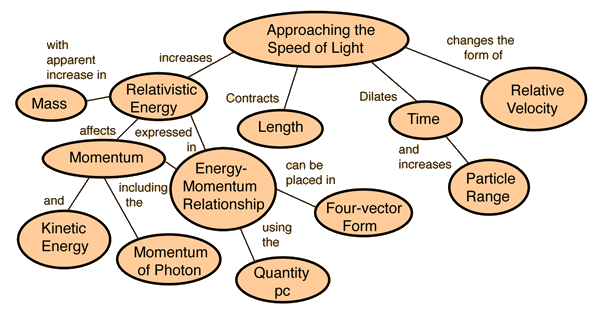At what energies must relativistic expressions be used?
Index

 HyperPhysics***** Relativity R Nave
Go Back

# Relativistic Momentum

The relativistic momentum is given bywhich is the ordinary definition of momentum with the mass replaced by the relativistic mass.

 For v = c, gamma = , and rest mass
 m0 = x10^kg = me= mp
 m0 = MeV/c2 = GeV/c2

the relativistic momentum is

 p = x10^kg m/s = MeV/c = GeV/c
compared to the non-relativistic result p = mv = x10^kg m/s
which would then be in error by %.

In the above calculations, one of the ways of expressing mass and momentum is in terms of electron volts. It is typical in high energy physics, where relativistic quantities are encountered, to make use of the Einstein relationship to relate mass and momentum to energy. In relativistic mechanics, the quantity pc is often used in momentum discussions. It has the units of energy.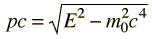For extreme relativistic velocities wherethenA useful application of the quantity pc is in the calculation of the velocity as a fraction of c.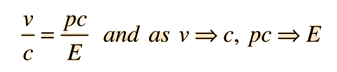and as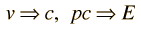with the limiting case applying for the momentum of a photon.
 Momentum of photon Usefulness of the quantity pc
Index

 HyperPhysics***** Relativity R Nave
Go Back

# Momentum of Photon

For a photon, the relativistic momentum expressionapproaches zero over zero, so it can't be used directly to determine the momentum of a zero rest mass particle. But the general energy expression can be put in the form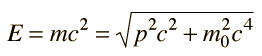and by setting rest mass equal to zero and applying the Planck relationship, we get the momentum expression: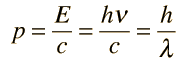Wave-particle duality application
Index

 HyperPhysics***** Relativity R Nave
Go Back

# Relativistic Energy in Terms of Momentum

The famous Einstein relationship for energy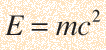can be blended with the relativistic momentum expression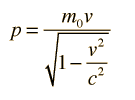to give an alternative expression for energy.
The combination pc shows up often in relativistic mechanics. It can be manipulated as follows: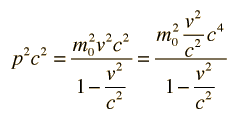and by adding and subtracting a term it can be put in the form: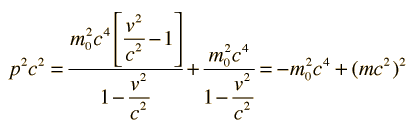which may be rearranged to give the expression for energy:Note that the m with the zero subscript is the rest mass, and that m without a subscript is the effective relativistic mass.
Index

 HyperPhysics***** Relativity R Nave
Go Back